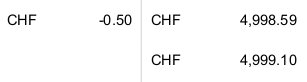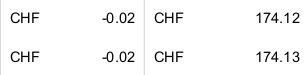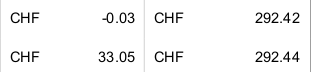# How to deal with money in software

Date 2020-07-28

I recently did my accounting for my investment account. I noticed a significant amount of errors in the account statement that my broker gave me.

After a bit of a rant on Twitter, I want to explain what they likely did wrong and how to deal with money correctly in software.

## The issue

While doing my accounting, I noticed about ten mistakes of the following kind over a timespan of less than two years:

• $4999.10 - 0.50$ is $4998.59$ apparently:• $174.13 - 0.02$ is $174.12$ apparently:• $292.44 - 0.03$ is $292.42$ apparently:## Disclaimer

I have contacted my broker about this privately, and asked them to confirm that they are not using IEEE754 floating point numbers for calculations involving money. They refused to confirm that they are not using IEEE754 floating point numbers for calculations involving money. They have also explicitly stated that "All entries on your account are rounded to the nearest cent." and they have made it clear that they do not see this as a problem. I have also offered my services, as someone who has experience with getting things like this exactly right, but I did not get a response to that offer.

If you have only come here to get outraged about how my broker does not care about the correctness of their software and their accounting, you can stop reading now. The rest of this blogpost will be a detailed explanation of the problems at hand and how to solve them.

## Problems with money

Because computers are really good at doing computations, you might think that they must therefore be good at dealing with money. However, money is a very complex subject. There are all sorts of pitfalls, and this list of falsehoods is a good place to start learning about them.

### Money as a floating point value

If you take away one thing from this blogpost, let it be this:

You must NOT store monetary values as IEEE 754 floating point numbers.

Corollary: Given that this is the only numeric format that JavaScript has, this implies that you must not use JavaScript for calculations with currency.

These are the primary reasons:

1. Calculations involving floating point numbers usually have some amount of error.
2. Many floating point numbers do not make sense as a value of money.

### Creating money out of thin air using floating point calculations

Floating point number calculations usually have some error. As an example, let us have a look at the value $0.1$, which cannot represented accurately using an IEEE754 floating point number. You can tell this by looking at 0.1 + 0.1 + 0.1:

λ> 0.1 + 0.1 + 0.1
0.30000000000000004


This means that when you get a dime from a customer three times, and store it as a floating number, you will have effectively created 0.00000000000000004 USD out of thin air. This might not seem like much (even though it does not need to be much, it is still wrong), but this error grows as the values in your calculations grow. There is an entire field of mathematics dedicated to thinking about what happens to the errors depending on what types of calculations you are performing.

### Representing nonsense values of money

The IEEE754 Floating point number spec defines NaN, +Infinity and -Infinity. These make no sense when interpreting them as monetary values. I have to mention this because these values form a real problem in practice.

You might be thinking: OK, but I take good care not to run into issues with these non-normalised floating point values. You are still wrong, but let us assume you are not. You will still run into the next problem

#### Minimal quantisations

The smallest denomination of US dollar that you can hold or transact is 0.01 USD: a penny. Any amount of US dollars more granular than that does not exist in coins and cannot usually be transacted. (You can still potentially have more granular debts, as you will see below.)

Even worse: different currencies have different minimal quantisations. In Switzerland, where I am using my broker, the smallest coin of a Swiss Frank is 5 "rappen": 0.05 CHF. The transaction granularity goes down to 0.01 CHF in bank transactions but no lower than that either.

## Solutions

### Requirements

In order to begin talking about solutions, let us clarify what needs to be possible in the system that we produce:

1. We must be able to add up amounts of money.

This is useful to keep a running balance, for example.

The additions must happen in such a way that the representation of the sum equals the sum of the representations. Otherwise we might create or destroy money via addition.

For example, 0.1 + 0.1 + 0.1 must somehow equal 0.3 and not 0.30000000000000004.

#### Integral Multiplication

1. We must be able to multiply amounts of money by an integer.

This is useful to find out how much multiple units of an item would cost, in the case of integral multiples.

You will notice that if you can solve the problem of addition, then you have also solved the problem of correct integral multiplication However, ideally, an integral multiplication would take up the same amount of time as a single addition, not a linear multiple of it. We would like to have multiplication be efficient as well as correct.

For example, 3.33 x 3 must equal 9.99 and not 10.

#### Division by an integer

1. We must be able to divide an amount of money by an integer.

This is useful for dividing a payment into installments. Here it is important that the sum of the installments equals the amount that was divided.

For example, 10 / 3 must be divided into three times 3.33 and an extra 0.01 somewhere: 3.34 + 3.33 + 3.33.

#### Fractional Multiplication

1. We must be able to multiply amounts of money by a fraction.

This is useful for calculating interest rates, taxes and dividends, for example.

It should happen in such a way that the representation of the product equals the product of the representations. Failing that (because we'll see that this is impossible), it should at least not create or destroy money.

For example, 0.1 times 0.11 USD, using a minimal quantisation of 0.01 USD, should result in 0.01 USD. The representational error which mathematically equals 0.001 should be dealt with somehow.

#### Accurate Accounting

1. We must be able to accurately account for every smallest unit of currency.

When earning or spending money, we must be able to account for every smallest unit of currency in play. No such units be created or destroyed, and no amounts smaller than the smallest unit may be accounted for.

Note that you can choose a different minimal quantisation of currency in your accounting system than is used for bank transactions. Even if banks use 0.01 CHF as a minimal transaction value, you can still use 0.0001 CHF as a minimal quantisation internally. The only real constraints are correctness: you do not create or destroy money and storage size. Dealing with more precise numbers requires more storage.

When you choose a different minimal quantisation of currency internally than the one you show to users, you have to somehow make that conversion. When you do, it is important that you don't add any mistakes back in.

This is likely what my broker did wrong. They probably used rounding from 4 decimals (a common standard) to 2 decimals and introduced rounding errors in the process.

#### Granular pricing

1. We must be able to make calculations with values smaller than the smallest unit of currency

The utility of this requirement may not be obvious. However, when dealing with an item of large volume and cost such as oil, we want to be able to reason about (and compete on) the (average) price of a small amount of it. In such a case it may be necessary to reason about currency in units smaller than its minimal quantisation.

For example, if I want to rent out digital storage for $0.000023$ USD per kilobyte per month, that rate is like expressed in a more precise granularity then your system deals with. In that case we must still be able to rent out $2'000'000$ kilobyte for a month for 46.00 USD.

### Representation

It is already clear that we must not represent currency as floating point numbers. Instead, we must use a representation with infinite precision: integers.

For the purposes of all but the special case of 'granular pricing', we must use integers to represent currency. For each individual currency we will represent an amount of it as an integral number of its smallest units.

For example, 1 USD could be represented as 100 at a minimal quantisation of 0.01 USD because 100 x 0.01 USD = 1 USD and 1 CHF could be represented as 20 at a minimal quantisation of 0.05 CHF because 20 x 0.05 CHF = 1 CHF. If you choose a smaller minimal quantisation, such as 0.0001 CHF, then you would represent 1 CHF as 1000 instead. Given that the minimal quantisation is not obvious, make sure to document it well (and/or put it on the type-level).

In an ideal world you would use arbitrarily-sized integers, but often you may be forced to use bounded representations with fixed limits. It is possible to accommodate such constraints but the trade-offs, as you will read next, are real and should be considered carefully. In what follows we will continue by assuming you are using (bounded-size) integer numbers.

Addition of integers is relatively simple and well supported. However, most implementations of addition are not suitable for use with fixed-sized integer representations. Indeed, most implementations of addition will just roll over around the bounds.

For example, consider a situation in which you are a bank and you store amounts of money as int32. A very wealthy client of yours has about twenty million dollars. You save this information as "this client has two billion cents". A bit later, he earns another two million dollars, but when the money is sent to him, suddenly the system says that he is millions in of dollars in debt. There are two big issues with this scenario: 1. The client has lost money and 2. The total amount of money has decreased:

λ> 2000000000 + 200000000 :: Int32
-2094967296


Instead, we must use an implementation of addition that can deal with overflow safely (see my recommendations below).

### Integer division

When dividing an amount of money by an integer, we have the problem of remainders.

As an example, let us look at a payment of 1000.00 CHF that needs to be paid in three installments. Let's assume we chose a minimal quantisation of 0.05 CHF. Mathematically dividing $1000.00$ by $3$ yields $333.33..$ repeated. However, 0.03 CHF does not exist when using this minimal quantisation and repeating fractions definitely do not, so what do we do?

In practice, we should round down the result to the nearest existing amount of currency. In this case that is 333.30 CHF. Then we need to calculate the remainder, which is 1000.00 CHF - 3 x 333.30 CHF = 0.10 CHF and decide what to do with it.

You could assign the remainder to any of the installments to get 333.40 CHF, 333.30 CHF and 333.30 CHF. You could also divide the remainder among the installments to get 333.35 CHF, 333.35 CHF and 333.30 CHF. This part is up to you, but as long as you deal with the remainder, you should end up with accurate accounting.

### Fractional multiplication, accurate accounting and granular pricing

As long as we only perform addition and integer multiplication, the accurate accounting requirement is already fulfilled. It also turns out that if we can solve fractional multiplication in an accurate way, that we can get granular pricing for free.

The big issue with fractional multiplication is easily illustrated with currency conversions. For example, as of the time of writing, the conversion rate from EUR to CHF is 1 EUR = 1.072032 CHF. At a first glance this really does not make sense because 1.072032 CHF really does not mean anything if we choose a minimal quantisation greater than 0.0000001 CHF. (Note that there will always be rates that don't make sense for your minimal quantisation, so you have to deal with this problem.) There exists 1.05 CHF and 1.10 CHF but there can never be 1.072032 CHF using this minimal quantisation. However, you never really convert exactly one EUR either. This is the important piece of info that will allow us to solve the problem.

You cannot simply multiply 1 EUR by 1.072032 CHF/EUR to get a sensible value in CHF. Instead, a bit more infrastructure is needed. The way you should interpret such a ratio is a bit different from your intuition.

To exchange 10 000 EUR to CHF, we first make a hypothetical calculation of what would happen if currencies had no minimal quantisation:

10 000.00 EUR * 1.072032 CHF / EUR =  10 720.32 CHF


Next, we choose the closest meaningful value to the result. Recall that we chose a minimal quantisation of 0.05 CHF, so the closest meaningful value is 10 720.30 CHF. We represent this as the integer 214406 because 214406 * 0.05 CHF = 10 720.30 CHF.

You now change the rate that you give so that this matches nicely, and represent the rate as an integral fraction:

214 406 % 10 000 00 = 107 203 % 5 000 00

We represent only the conversion rate as a fraction, not amounts of money. Note that you have to represent this as two numbers in memory, because there is no guarantee that the result would not have unrepresentable repeating digits. Note also that the original rate only differs from this rate by 0.000002, and you can charge a higher rate if this is a problem for you. The 'error' also shrinks as transactions get bigger and the absolute (theoretical) error is never bigger than your chosen minimal quantisation so this is really no big deal in practice.

You can then safely use this rate to accurately convert 10 000.00 EUR to 10 720.32 CHF.

### Showing amounts to users

When you chose amounts to users, we need to make sure that they make sense. It should not look like we made any errors.

By far the simplest way to deal with this problem is to show amounts to users at the same level of granularity as we are storing them. Otherwise you may get errors like the ones my broker is showing me. You can still give users the option to show amounts in a more conventional way if you do not really care about these apparent mistakes.

## Libraries

I recommend safe-decimal for the purpose of storing amounts in Haskell. It allows you to force yourself to deal with arithmetic exceptions safely and choose an underlying integer type. It also deals with integer multiplication efficiently, as we had hoped for.

I cannot recommend safe-money as is because:

• It only deals with arbitrarily-sized integers.
• It has a concept of 'dense' currencies, which is misleading.

However, the idea of putting the currency and the minimal quantisation on the type-level is potentially great.

## Conclusions

It is possible to deal with currency accurately, but it is not easy. Listen to experts on this matter, and test your implementation well. You can use property-based testing, and validity-based testing in particular, to help you find problems with your implementation.

Self-management with Smos: Archiving

Know a technical team that could use strong technical leadership?

Introduction Self-management with Smos: the waiting-action report